Publicité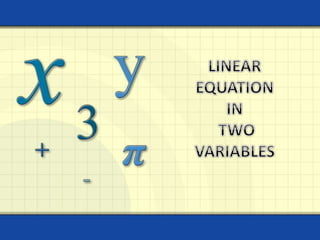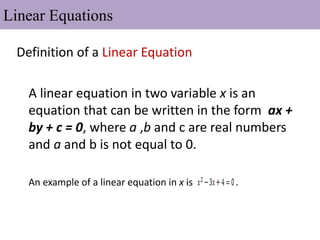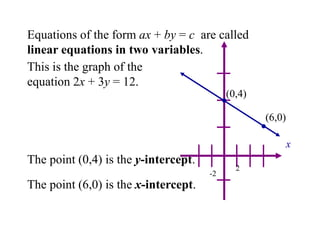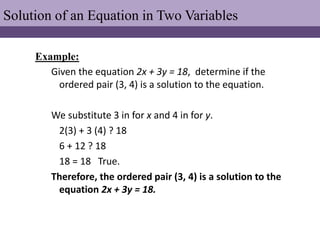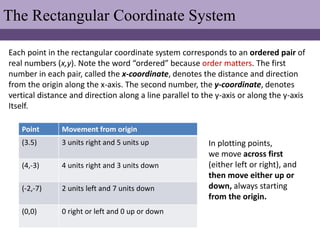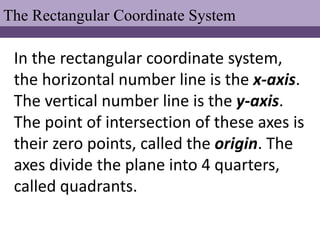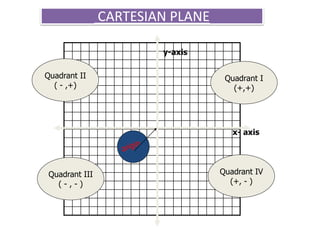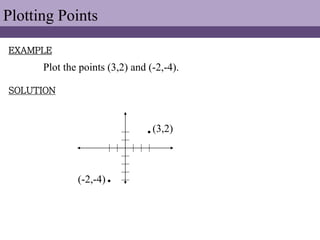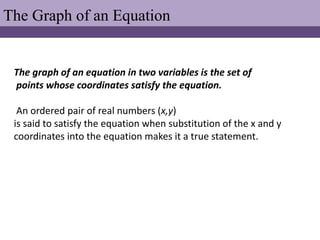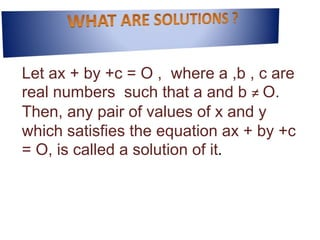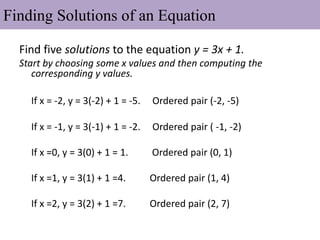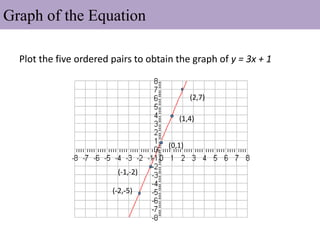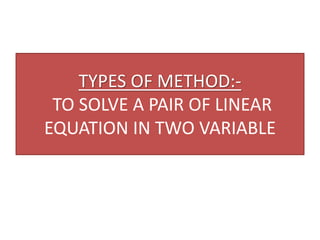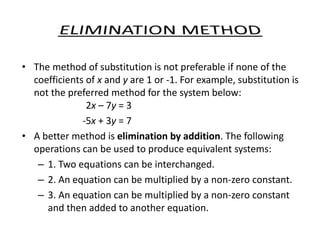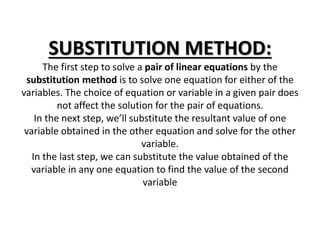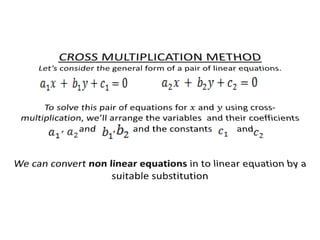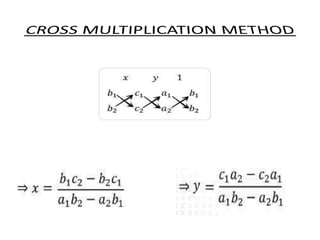1 sur 19
Publicité

### linear equation in 2 variables

1. Linear Equation in Two Variables
2. Linear Equations Definition of a Linear Equation A linear equation in two variable x is an equation that can be written in the form ax + by + c = 0, where a ,b and c are real numbers and a and b is not equal to 0. An example of a linear equation in x is .
3. Equations of the form ax + by = c are called linear equations in two variables. The point (0,4) is the y-intercept. The point (6,0) is the x-intercept. x 2 -2 This is the graph of the equation 2x + 3y = 12. (0,4) (6,0)
4. Example: Given the equation 2x + 3y = 18, determine if the ordered pair (3, 4) is a solution to the equation. We substitute 3 in for x and 4 in for y. 2(3) + 3 (4) ? 18 6 + 12 ? 18 18 = 18 True. Therefore, the ordered pair (3, 4) is a solution to the equation 2x + 3y = 18. Solution of an Equation in Two Variables
5. The Rectangular Coordinate System Each point in the rectangular coordinate system corresponds to an ordered pair of real numbers (x,y). Note the word “ordered” because order matters. The first number in each pair, called the x-coordinate, denotes the distance and direction from the origin along the x-axis. The second number, the y-coordinate, denotes vertical distance and direction along a line parallel to the y-axis or along the y-axis Itself. In plotting points, we move across first (either left or right), and then move either up or down, always starting from the origin. Point Movement from origin (3.5) 3 units right and 5 units up (4,-3) 4 units right and 3 units down (-2,-7) 2 units left and 7 units down (0,0) 0 right or left and 0 up or down
6. The Rectangular Coordinate System In the rectangular coordinate system, the horizontal number line is the x-axis. The vertical number line is the y-axis. The point of intersection of these axes is their zero points, called the origin. The axes divide the plane into 4 quarters, called quadrants.
7. CARTESIAN PLANE x- axis y-axis Quadrant I (+,+) Quadrant II ( - ,+) Quadrant IV (+, - ) Quadrant III ( - , - )
8. Plotting Points EXAMPLE SOLUTION Plot the points (3,2) and (-2,-4).   (3,2) (-2,-4)
9. The Graph of an Equation The graph of an equation in two variables is the set of points whose coordinates satisfy the equation. An ordered pair of real numbers (x,y) is said to satisfy the equation when substitution of the x and y coordinates into the equation makes it a true statement.
10. Let ax + by +c = O , where a ,b , c are real numbers such that a and b ≠ O. Then, any pair of values of x and y which satisfies the equation ax + by +c = O, is called a solution of it.
11. Find five solutions to the equation y = 3x + 1. Start by choosing some x values and then computing the corresponding y values. If x = -2, y = 3(-2) + 1 = -5. Ordered pair (-2, -5) If x = -1, y = 3(-1) + 1 = -2. Ordered pair ( -1, -2) If x =0, y = 3(0) + 1 = 1. Ordered pair (0, 1) If x =1, y = 3(1) + 1 =4. Ordered pair (1, 4) If x =2, y = 3(2) + 1 =7. Ordered pair (2, 7) Finding Solutions of an Equation
12. Plot the five ordered pairs to obtain the graph of y = 3x + 1 (2,7) (1,4) (0,1) (-1,-2) (-2,-5) Graph of the Equation
13. TYPES OF METHOD:- TO SOLVE A PAIR OF LINEAR EQUATION IN TWO VARIABLE
14. • The method of substitution is not preferable if none of the coefficients of x and y are 1 or -1. For example, substitution is not the preferred method for the system below: 2x – 7y = 3 -5x + 3y = 7 • A better method is elimination by addition. The following operations can be used to produce equivalent systems: – 1. Two equations can be interchanged. – 2. An equation can be multiplied by a non-zero constant. – 3. An equation can be multiplied by a non-zero constant and then added to another equation.
15. SUBSTITUTION METHOD: The first step to solve a pair of linear equations by the substitution method is to solve one equation for either of the variables. The choice of equation or variable in a given pair does not affect the solution for the pair of equations. In the next step, we’ll substitute the resultant value of one variable obtained in the other equation and solve for the other variable. In the last step, we can substitute the value obtained of the variable in any one equation to find the value of the second variable
Publicité